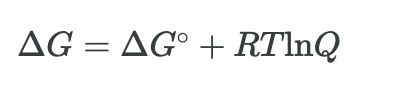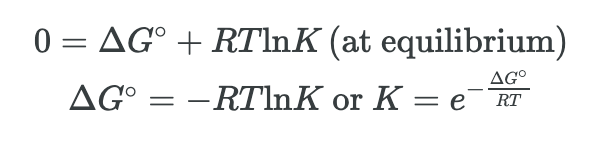Complimentary 1-hour tutoring consultation

MCAT Content / Rate Processes In Chemical Reactions Kinetics And Equilibrium / Relationship Of The Equilibrium Constant And Delta G

### Relationship of the Equilibrium Constant and ΔG°

Topic: Rate Processes In Chemical Reactions Kinetics And Equilibrium

When the reaction quotient is lesser than the equilibrium constant, a chemical reaction will proceed in the forward direction until equilibrium is reached and Q = K; however, if Q < K, the process will proceed in the reverse direction until equilibrium is achieved.

The free energy change for a process may be viewed as a measure of its driving force. A negative value for ΔG represents a finite driving force for the process in the forward direction, while a positive value represents a driving force for the process in the reverse direction. When ΔG is zero, the forward and reverse driving forces are equal, and so the process occurs in both directions at the same rate (the system is at equilibrium).

The reaction quotient, Q, is a measure of the status of an equilibrium system. When Q is lesser than the equilibrium constant, K, the reaction will proceed in the forward direction until equilibrium is reached and Q = K. Conversely, if Q < K, the process will proceed in the reverse direction until equilibrium is achieved.

The free energy change for a process taking place with reactants and products present under nonstandard conditions, ΔG, is related to the standard free energy change, ΔG°, according to this equation:R is the gas constant (8.314 J/K mol), T is the kelvin or absolute temperature, and Q is the reaction quotient.

For a system at equilibrium, Q = K and ΔG = 0, and the previous equation may be written asThis form of the equation provides a useful link between these two essential thermodynamic properties, and it can be used to derive equilibrium constants from standard free energy changes and vice versa.

The table below summarizes the relationship between G and the equilibrium constant K:

 K ΔG° Comments >1 <0 More products at equilibrium <1 >0 Reactants more abundant at equilibrium =1 =0 Equilibrium established equal amounts of products and reactants

Practice Questions

The thermodynamics of ATP hydrolysis in living cells

MCAT Official Prep (AAMC)

Section Bank C/P Section Question 24

Practice Exam 3 C/P Section Passage 8 Question 41

Practice Exam 3 C/P Section Passage 10 Question 55

Key Points

• Gibbs’s free energy and equilibrium can be related through the concept Q which is the reaction quotient and examines the progress of a reaction.

• The free energy change for a process may be viewed as a measure of its driving force. A negative value for ΔG represents a finite driving force for the process in the forward direction, while a positive value represents a driving force for the process in the reverse direction.

• When ΔG is zero, the forward and reverse driving forces are equal, and so the process occurs in both directions at the same rate (the system is at equilibrium).

• The equilibrium value can be calculated by setting ΔG = 0 when equilibrium has been established.

• Gibbs free energy and K, the equilibrium constant, can be used to determine the progress of a reaction.

• The reaction quotient, Q, is a measure of the status of an equilibrium system. When Q is lesser than the equilibrium constant, K, the reaction will proceed in the forward direction until equilibrium is reached and Q = K. Conversely, if Q < K, the process will proceed in the reverse direction until equilibrium is achieved.

Key Terms

Gibbs free energy change (G): thermodynamic property defined in terms of system enthalpy and entropy; all spontaneous processes involve a decrease in G.

Standard free energy change (ΔG°): change in free energy for a process occurring under standard conditions (1 bar pressure for gases, 1 M concentration for solutions)

Standard free energy of formation : change in free energy accompanying the formation of one mole of substance from its elements in their standard states

Reaction quotient (Q): Measures the relative amounts of products and reactants present during a reaction at a particular point in time.

Equilibrium constant (K): A number that expresses the relationship between the amounts of products and reactants present at equilibrium in a reversible chemical reaction at a given temperature.

Billing Information